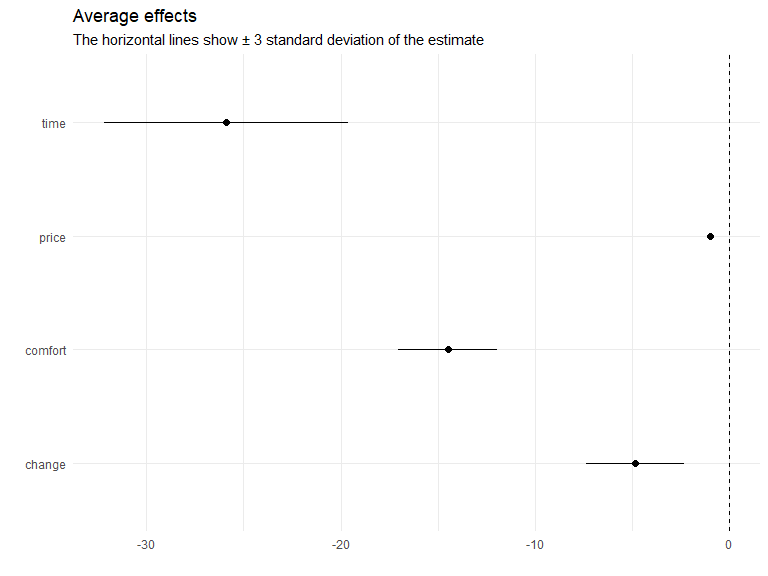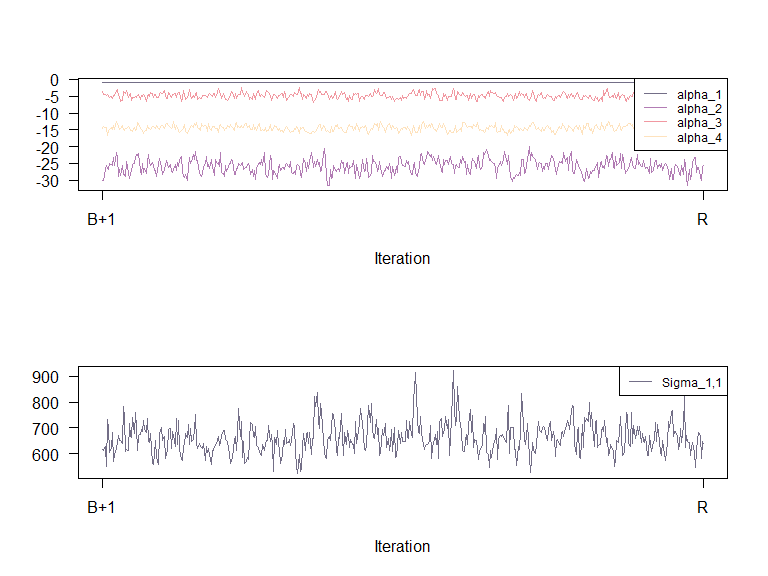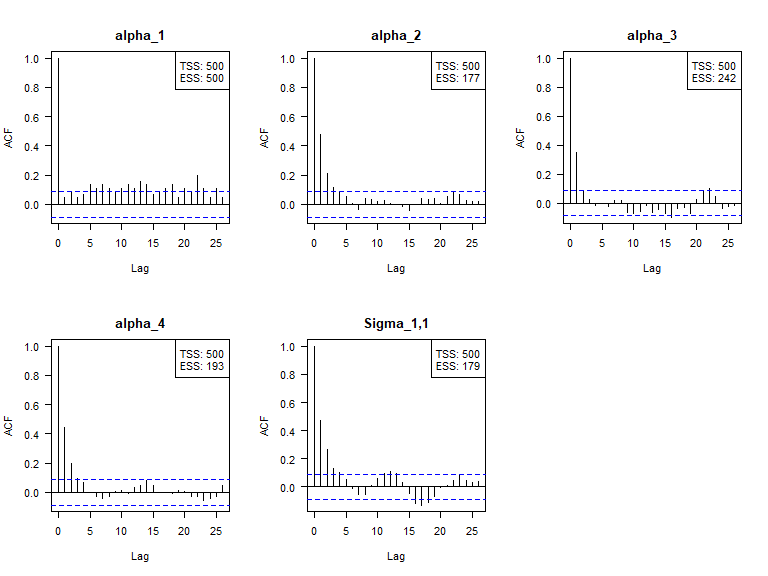# Model fitting

This vignette1 is a documentation of the estimation procedure fit_model() in {RprobitB}.

## Bayes estimation of the probit model

Bayes estimation of the probit model builds upon the work of McCulloch and Rossi (1994), Nobile (1998), Allenby and Rossi (1998), and Imai and Dyk (2005). A key ingredient is the concept of data augmentation, see Albert and Chib (1993): The idea is to treat the latent utilities $$U$$ in the model equation $$U = X\beta + \epsilon$$ as additional parameters. Then, conditional on $$U$$, the probit model constitutes a standard Bayesian linear regression set-up. Its posterior distribution can be approximated by iteratively drawing and updating each model parameter conditional on the other parameters (the so-called Gibbs sampling approach).

A priori, we assume the following (conjugate) parameter distributions:

• $$(s_1,\dots,s_C)\sim D_C(\delta)$$, where $$D_C(\delta)$$ denotes the $$C$$-dimensional Dirichlet distribution with concentration parameter vector $$\delta = (\delta_1,\dots,\delta_C)$$,

• $$\alpha\sim \text{MVN}_{P_f}(\psi,\Psi)$$, where $$\text{MVN}_{P_f}$$ denotes the $$P_f$$-dimensional normal distribution with mean $$\psi$$ and covariance $$\Psi$$,

• $$b_c \sim \text{MVN}_{P_r}(\xi,\Xi)$$, independent for all $$c$$,

• $$\Omega_c \sim W^{-1}_{P_r}(\nu,\Theta)$$, independent for all $$c$$, where $$W^{-1}_{P_r}(\nu,\Theta)$$ denotes the $$P_r$$-dimensional inverse Wishart distribution with $$\nu$$ degrees of freedom and scale matrix $$\Theta$$,

• and $$\Sigma \sim W^{-1}_{J-1}(\kappa,\Lambda)$$.

These prior distributions imply the following conditional posterior distributions:

• The class weights are drawn from the Dirichlet distribution $\begin{equation} (s_1,\dots,s_C)\mid \delta,z \sim D_C(\delta_1+m_1,\dots,\delta_C+m_C), \end{equation}$ where for $$c=1,\dots,C$$, $$m_c=\#\{n:z_n=c\}$$ denotes the current absolute class size.2

• Independently for all $$n$$, we update the allocation variables $$(z_n)_n$$ from their conditional distribution $\begin{equation} \text{Prob}(z_n=c\mid s,\beta,b,\Omega )=\frac{s_c\phi_{P_r}(\beta_n\mid b_c,\Omega_c)}{\sum_c s_c\phi_{P_r}(\beta_n\mid b_c,\Omega_c)}. \end{equation}$

• The class means $$(b_c)_c$$ are updated independently for all $$c$$ via $\begin{equation} b_c\mid \Xi,\Omega,\xi,z,\beta \sim\text{MVN}_{P_r}\left( \mu_{b_c}, \Sigma_{b_c} \right), \end{equation}$ where $$\mu_{b_c}=(\Xi^{-1}+m_c\Omega_c^{-1})^{-1}(\Xi^{-1}\xi +m_c\Omega_c^{-1}\bar{b}_c)$$, $$\Sigma_{b_c}=(\Xi^{-1}+m_c\Omega_c^{-1})^{-1}$$, $$\bar{b}_c=m_c^{-1}\sum_{n:z_n=c} \beta_n$$.

• The class covariance matrices $$(\Omega_c)_c$$ are updated independently for all $$c$$ via $\begin{equation} \Omega_c \mid \nu,\Theta,z,\beta,b \sim W^{-1}_{P_r}(\mu_{\Omega_c},\Sigma_{\Omega_c}), \end{equation}$ where $$\mu_{\Omega_c}=\nu+m_c$$ and $$\Sigma_{\Omega_c}=\Theta^{-1} + \sum_{n:z_n=c} (\beta_n-b_c)(\beta_n-b_c)'$$.

• Independently for all $$n$$ and $$t$$ and conditionally on the other components, the utility vectors $$(U_{nt:})$$ follow a $$J-1$$-dimensional truncated multivariate normal distribution, where the truncation points are determined by the choices $$y_{nt}$$. To sample from a truncated multivariate normal distribution, we apply a sub-Gibbs sampler, following the approach of Geweke (1998): $\begin{equation} U_{ntj} \mid U_{nt(-j)},y_{nt},\Sigma,W,\alpha,X,\beta \sim \mathcal{N}(\mu_{U_{ntj}},\Sigma_{U_{ntj}}) \cdot \begin{cases} 1(U_{ntj}>\max(U_{nt(-j)},0) ) & \text{if}~ y_{nt}=j\\ 1(U_{ntj}<\max(U_{nt(-j)},0) ) & \text{if}~ y_{nt}\neq j \end{cases}, \end{equation}$ where $$U_{nt(-j)}$$ denotes the vector $$(U_{nt:})$$ without the element $$U_{ntj}$$, $$\mathcal{N}$$ denotes the univariate normal distribution, $$\Sigma_{U_{ntj}} = 1/(\Sigma^{-1})_{jj}$$ and $\begin{equation} \mu_{U_{ntj}} = W_{ntj}'\alpha + X_{ntj}'\beta_n - \Sigma_{U_{ntj}} (\Sigma^{-1})_{j(-j)} (U_{nt(-j)} - W_{nt(-j)}'\alpha - X_{nt(-j)}' \beta_n ), \end{equation}$ where $$(\Sigma^{-1})_{jj}$$ denotes the $$(j,j)$$th element of $$\Sigma^{-1}$$, $$(\Sigma^{-1})_{j(-j)}$$ the $$j$$th row without the $$j$$th entry, $$W_{nt(-j)}$$ and $$X_{nt(-j)}$$ the coefficient matrices $$W_{nt}$$ and $$X_{nt}$$, respectively, without the $$j$$th column.

• Updating the fixed coefficient vector $$\alpha$$ is achieved by applying the formula for Bayesian linear regression of the regressors $$W_{nt}$$ on the regressands $$(U_{nt:})-X_{nt}'\beta_n$$, i.e. $\begin{equation} \alpha \mid \Psi,\psi,W,\Sigma,U,X,\beta \sim \text{MVN}_{P_f}(\mu_\alpha,\Sigma_\alpha), \end{equation}$ where $$\mu_\alpha = \Sigma_\alpha (\Psi^{-1}\psi + \sum_{n=1,t=1}^{N,T} W_{nt} \Sigma^{-1} ((U_{nt:})-X_{nt}'\beta_n) )$$ and $$\Sigma_\alpha = (\Psi^{-1} + \sum_{n=1,t=1}^{N,T} W_{nt}\Sigma^{-1} W_{nt}^{'} )^{-1}$$.

• Analogously to $$\alpha$$, the random coefficients $$(\beta_n)_n$$ are updated independently via $\begin{equation} \beta_n \mid \Omega,b,X,\Sigma,U,W,\alpha \sim \text{MVN}_{P_r}(\mu_{\beta_n},\Sigma_{\beta_n}), \end{equation}$ where $$\mu_{\beta_n} = \Sigma_{\beta_n} (\Omega_{z_n}^{-1}b_{z_n} + \sum_{t=1}^{T} X_{nt} \Sigma^{-1} (U_{nt:}-W_{nt}'\alpha) )$$ and $$\Sigma_{\beta_n} = (\Omega_{z_n}^{-1} + \sum_{t=1}^{T} X_{nt}\Sigma^{-1} X_{nt}^{'} )^{-1}$$ .

• The error term covariance matrix $$\Sigma$$ is updated by means of $\begin{equation} \Sigma \mid \kappa,\Lambda,U,W,\alpha,X,\beta \sim W^{-1}_{J-1}(\kappa+NT,\Lambda+S), \\ \end{equation}$ where $$S = \sum_{n=1,t=1}^{N,T} \varepsilon_{nt} \varepsilon_{nt}'$$ and $$\varepsilon_{nt} = (U_{nt:}) - W_{nt}'\alpha - X_{nt}'\beta_n$$.

### Parameter normalization

Samples obtained from the updating scheme described above lack identification (except for $$s$$ and $$z$$ draws), compare to the vignette on the model definition. Therefore, subsequent to the sampling, the following normalizations are required for the $$i$$th updates in each iterations $$i$$:

• $$\alpha^{(i)} \cdot \omega^{(i)}$$,

• $$b_c^{(i)} \cdot \omega^{(i)}$$, $$c=1,\dots,C$$,

• $$U_{nt}^{(i)} \cdot \omega^{(i)}$$, $$n = 1,\dots,N$$, $$t = 1,\dots,T$$,

• $$\beta_n^{(i)} \cdot \omega^{(i)}$$, $$n = 1,\dots,N$$,

• $$\Omega_c^{(i)} \cdot (\omega^{(i)})^2$$, $$c=1,\dots,C$$, and

• $$\Sigma^{(i)} \cdot (\omega^{(i)})^2$$,

where either $$\omega^{(i)} = \sqrt{\text{const} / (\Sigma^{(i)})_{jj}}$$ with $$(\Sigma^{(i)})_{jj}$$ the $$j$$th diagonal element of $$\Sigma^{(i)}$$, $$1\leq j \leq J-1$$, or alternatively $$\omega^{(i)} = \text{const} / \alpha^{(i)}_p$$ for some coordinate $$1\leq p \leq P_f$$ of the $$i$$th draw for the coefficient vector $$\alpha$$. Here, $$\text{const}$$ is any positive constant (typically 1). The preferences will be flipped if $$\omega^{(i)} < 0$$, which only is the case if $$\alpha^{(i)}_p < 0$$.

### Burn-in and thinning

The theory behind Gibbs sampling constitutes that the sequence of samples produced by the updating scheme is a Markov chain with stationary distribution equal to the desired joint posterior distribution. It takes a certain number of iterations for that stationary distribution to be approximated reasonably well. Therefore, it is common practice to discard the first $$B$$ out of $$R$$ samples (the so-called burn-in period). Furthermore, correlation between nearby samples should be expected. In order to obtain independent samples, we consider only every $$Q$$th sample when computing Gibbs sample statistics like expectation and standard deviation. The independence of the samples can be verified by computing the serial correlation and the convergence of the Gibbs sampler can be checked by considering trace plots, see below.

## The fit_model() function

The Gibbs sampling scheme described above can be executed by applying the function

fit_model(data = data)

where data must be an RprobitB_data object (see the vignette about choice data). The function has the following optional arguments:

• scale: A character which determines the utility scale. It is of the form "<parameter> := <value>", where <parameter> is either the name of a fixed effect or Sigma_<j>,<j> for the <j>th diagonal element of Sigma, and <value> is the value of the fixed parameter (i.e. $$\text{const}$$ introduced above). Per default scale = "Sigma\_1,1 := 1", i.e. the first error-term variance is fixed to 1.

• R: The number of iterations of the Gibbs sampler. The default is R = 10000.

• B: The length of the burn-in period, i.e. a non-negative number of samples to be discarded. The default is B = R/2.

• Q: The thinning factor for the Gibbs samples, i.e. only every Qth sample is kept. The default is Q = 1.

• print_progress: A boolean, determining whether to print the Gibbs sampler progress.

• prior: A named list of parameters for the prior distributions (their default values are documented in the check_prior() function):

• eta: The mean vector of length P_f of the normal prior for alpha.

• Psi: The covariance matrix of dimension P_f x P_f of the normal prior for alpha.

• delta: The concentration parameter of length 1 of the Dirichlet prior for s.

• xi: The mean vector of length P_r of the normal prior for each b_c.

• D: The covariance matrix of dimension P_r x P_r of the normal prior for each b_c.

• nu: The degrees of freedom (a natural number greater than P_r) of the Inverse Wishart prior for each Omega_c.

• Theta: The scale matrix of dimension P_r x P_r of the Inverse Wishart prior for each Omega_c.

• kappa: The degrees of freedom (a natural number greater than J-1) of the Inverse Wishart prior for Sigma.

• E: The scale matrix of dimension J-1 x J-1 of the Inverse Wishart prior for Sigma.

• latent_classes: A list of parameters specifying the number and the updating scheme of latent classes, see the vignette on modeling heterogeneity fitting.

## Example

In the previous vignette on choice data, we introduced the Train data set from the {mlogit} package that contains 2922 choices between two fictional train route alternatives. First, we transform the travel time from minutes to hours and the travel price from guilders to euros:

data("Train", package = "mlogit")
Train$price_A <- Train$price_A / 100 * 2.20371
Train$price_B <- Train$price_B / 100 * 2.20371
Train$time_A <- Train$time_A / 60
Train$time_B <- Train$time_B / 60

The following lines fit a probit model that explains the chosen trip alternatives (choice) by their price, time, number of changes, and level of comfort (the lower this value the higher the comfort). For normalization, the first linear coefficient, the price, was fixed to -1, which allows to interpret the other coefficients as monetary values:

form <- choice ~ price + time + change + comfort | 0
data <- prepare_data(form = form, choice_data = Train)
model_train <- fit_model(
data = data,
scale = "price := -1"
)

The estimated coefficients (using the mean of the Gibbs samples as a point estimate) can be printed via

coef(model_train)
#>            Estimate   (sd)
#> 1   price     -1.00 (0.00)
#> 2    time    -25.90 (2.09)
#> 3  change     -4.82 (0.84)
#> 4 comfort    -14.49 (0.86)

and visualized via

plot(coef(model_train), sd = 3)The results indicate that the deciders value one hour travel time by about 25€, an additional change by 5€, and a more comfortable class by 14€.3

## Checking the Gibbs samples

The Gibbs samples are saved in list form in the RprobitB_fit object at the entry "gibbs_samples", i.e.

str(model_train$gibbs_samples, max.level = 2, give.attr = FALSE) #> List of 2 #>$ gibbs_samples_raw:List of 2
#>   ..$alpha: num [1:1000, 1:4] -0.000713 -0.022961 -0.031988 -0.036215 -0.03571 ... #> ..$ Sigma: num [1:1000, 1] 1.05 1.1 1.03 1.02 1.01 ...
#>  $gibbs_samples_nbt:List of 2 #> ..$ alpha: num [1:500, 1:4] -1 -1 -1 -1 -1 -1 -1 -1 -1 -1 ...
#>   ..\$ Sigma: num [1:500, 1] 617 611 626 552 734 ...

This object contains 2 elements:

• gibbs_samples_raw is a list of the raw samples from the Gibbs sampler,

• and gibbs_samples_nbt are the Gibbs samples used for parameter estimates, i.e. the normalized and thinned Gibbs samples after the burn-in.

Calling the summary function on the estimated RprobitB_fit object yields additional information about the Gibbs samples gibbs_samples_nbt. You can specify a list FUN of functions that compute any point estimate of the Gibbs samples4, for example

• mean for the arithmetic mean,

• stats::sd for the standard deviation,

• R_hat for the Gelman-Rubin statistic 5,

• or custom statistics like the absolute difference between the median and the mean.

summary(model_train,
FUN = c("mean"        = mean,
"sd"          = stats::sd,
"R^"          = R_hat,
"custom_stat" = function(x) abs(mean(x) - median(x))
)
)
#> Probit model
#> Formula: choice ~ price + time + change + comfort | 0
#> R: 1000, B: 500, Q: 1
#> Level: Utility differences with respect to alternative 'B'.
#> Scale: Coefficient of effect 'price' (alpha_1) fixed to -1.
#>
#> Gibbs sample statistics
#>                mean           sd           R^  custom_stat
#>  alpha
#>
#>      1        -1.00         0.00         1.00         0.00
#>      2       -25.90         2.09         1.04         0.07
#>      3        -4.82         0.84         1.00         0.02
#>      4       -14.49         0.86         1.00         0.02
#>
#>  Sigma
#>
#>    1,1       661.69        59.21         1.03         7.30

Calling the plot method with the additional argument type = "trace" plots the trace of the Gibbs samples gibbs_samples_nbt:

par(mfrow = c(2,1))
plot(model_train, type = "trace")Additionally, we can visualize the serial correlation of the Gibbs samples via the argument type = "acf". The boxes in the top-right corner state the total sample size TSS (here R - B = 10000 - 5000 = 5000), the effective sample size ESS, and the factor by which TSS is larger than ESS.

par(mfrow = c(2,3))
plot(model_train, type = "acf")Here, the effective sample size is the value $$\text{TSS} / (1 + \sum_{k\geq 1} \rho_k)$$, where $$\rho_k$$ is the auto correlation between the chain offset by $$k$$ positions. The auto correlations are estimated via the stats::acf() function.

## Model transformation after estimation

The transform method can be used to transform an RprobitB_fit object in three ways:

1. change the length B of the burn-in period, for example
model_train <- transform(model_train, B = 1)
1. change the thinning factor Q of the Gibbs samples, for example
model_train <- transform(model_train, Q = 100)
1. or change the model normalization scale, for example
model_train <- transform(model_train, scale = "Sigma_1 := 1")

1. This vignette is built using R 4.2.1 with the {RprobitB} 1.1.2 package.↩︎

2. Mind that the model is invariant to permutations of the class labels $$1,\dots,C$$. For that reason, we accept an update only if the ordering $$s_1>\dots>s_C$$ holds, thereby ensuring a unique labeling of the classes.↩︎

3. These results are consistent with the ones that are presented in a vignette of the mlogit package on the same data set but using the logit model.↩︎

4. Use the function point_estimates() to access the Gibbs sample statistics as an RprobitB_parameter object.↩︎

5. A Gelman-Rubin statistic close to 1 indicates that the chain of Gibbs samples converged to the stationary distribution.↩︎